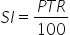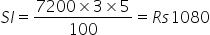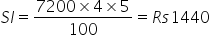Maths-
General
Easy

Question

# Equal sum of Rs. 7200 were lent to Ajay and Vijay at 5% per annum for a period of 3 years and 4 years respectively. What is the difference of the interest paid by Ajay and Vijay ?Hint:

## The correct answer is: Rs 360

### Complete step by step solution:We calculate simple interest by the formula,…(i)where P is Principal amount, T is number of years and R is rate of interestLet the sum of money = P = 7200 and Rate of interest R = 5%Case Ⅰ - AjayHere, we have T1 = 3.On substituting the values in (i), we get…(ii)Case Ⅱ - VijayHere, we have T1 = 4.On substituting the values in (i), we get…(iii)Difference of interest paid by Ajay and Vijay = 1440 - 1080 = Rs 360Vijay had to pay an extra of Rs 360 than Ajay.#### With Turito Foundation.#### Get an Expert Advice From Turito.